Click to Chat

1800-1023-196

+91-120-4616500

CART 0

• 0

MY CART (5)

Use Coupon: CART20 and get 20% off on all online Study Material

ITEM
DETAILS
MRP
DISCOUNT
FINAL PRICE
Total Price: Rs.

There are no items in this cart.
Continue Shopping• Complete JEE Main/Advanced Course and Test Series
• OFFERED PRICE: Rs. 15,900
• View Details

Congruence Exercise 16.2

Question: 1

In the following pairs of triangle (Figures), the lengths of the sides are indicated along sides. By applying SSS condition, determine which are congruent. State the result in symbolic.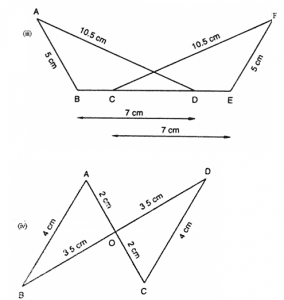Solution: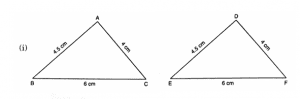1) In Δ ABC and Δ DEF

AB = DE = 4.5 cm (Side)

BC = EF = 6 cm (Side) and

AC = DF = 4 cm (Side)

Therefore, by SSS criterion of congruence, ΔABC ≅ ΔDEF

2)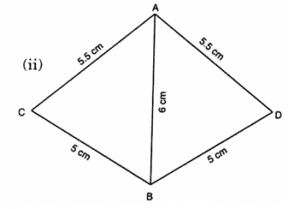In Δ ACB and Δ ADB

BC = BD (Side) and

AB = AB (Side)

Therefore, by SSS criterion of congruence, ΔACB ≅ ΔADB

3) In Δ ABD and Δ FEC,

AB = FE (Side)

BD = CE (Side)

Therefore, by SSS criterion of congruence, ΔABD ≅ ΔFEC

4) In Δ ABO and Δ DOC,

AB = DC (Side)

AO = OC (Side)

BO = OD (Side)

Therefore, by SSS criterion of congruence, ΔABO ≅ ΔODC

Question: 2

In figure, AD = DC and AB = BC

(i) Is ΔABD ≅ ΔCBD?

(ii) State the three parts of matching pairs you have used to answer (i).Solution:

Yes ΔABD = ΔCBD by the SSS criterion. We have used the three conditions in the SSS criterion as follows:

AB = BC and

DB = BD

Question: 3

In Figure, AB = DC and BC = AD.

(i) Is ΔABC ≅ ΔCDA?

(ii) What congruence condition have you used?

(iii) You have used some fact, not given in the question, what is that?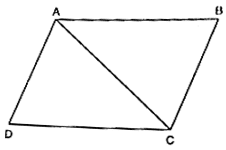Solution:

We have AB = DC

and AC = AC

Therefore by SSS ΔABC ≅ ΔCDA

We have used Side congruence condition with one side common in both the triangles.

Yes, have used the fact that AC = CA.

Question: 4

In ΔPQR ≅ ΔEFD,

(i) Which side of ΔPQR equals ED?

(ii) Which angle of ΔPQR equals angle E?

Solution:

ΔPQR ≅ ΔEFD

(i) Therefore PR = ED since the corresponding sides of congruent triangles are equal.

(ii) ∠QPR = ∠FED since the corresponding angles of congruent triangles are equal.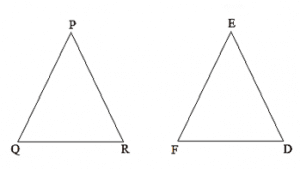Question: 5

Triangles ABC and PQR are both isosceles with AB = AC and PO = PR respectively. If also, AB = PQ and BC = QR, are the two triangles congruent? Which condition do you use?

It ∠B = 50°, what is the measure of ∠R?

Solution:

We have AB = AC in isosceles ΔABC

And PQ = PR in isosceles ΔPQR.

Also, we are given that AB = PQ and QR = BC.

Therefore, AC = PR (AB = AC, PQ = PR and AB = PQ)

Hence, ΔABC ≅ ΔPQR

Now

∠ABC = ∠PQR (Since triangles are congruent)However, ΔPQR is isosceles.

Therefore, ∠PRQ = ∠PQR = ∠ABC = 50°

Question: 6

ABC and DBC are both isosceles triangles on a common base BC such that A and D lie on the same side of BC. Are triangles ADB and ADC congruent? Which condition do you use? If ∠BAC = 40° and ∠BDC = 100°, then find ∠ADB.

Solution:

∠ABC + ∠BCA + ∠BAC = 180° (Angle sum property)

Since ΔABC is an isosceles triangle,

∠ABC = ∠BCA ∠ABC +∠ABC + 40°= 180°

2 ∠ABC = 180° – 40° = 140° ∠ABC = 140°/2 = 70°

∠DBC + ∠ BCD + ∠ BDC = 180° (Angle sum property)

Since ΔABC is an isosceles triangle, ∠ DBC = ∠BCD ∠DBC + ∠DBC + 100o = 180°

2 ∠DBC = 180° – 100o = 80°

∠DBC = 80°/2 = 40°

30° + 20° + ∠ADB = 180° (∠ADB = ∠ABC – ∠DBC), ∠ADB = 180°- 20° – 30°

Question: 7

Δ ABC and ΔABD are on a common base AB, and AC = BD and BC = AD as shown in Figure. Which of the following statements is true?

(i) ΔABC ≅ ΔABD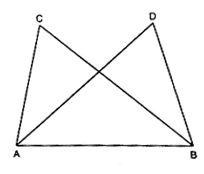Solution:

In ΔABC and ΔBAD we have,

AC = BD (given)

and AB = BA (common)

Therefore by SSS criterion of congruency, ΔABC ≅ ΔBAD

There option (iii) is true.

Question: 8

In Figure, ΔABC is isosceles with AB = AC, D is the mid-point of base BC.

(ii) State the three pairs of matching parts you use to arrive at your answer.Solution:

We have AB = AC.

Also since D is the midpoint of BC, BD = DC

Therefore by SSS condition,

We have used AB, AC : BD, DC AND AD, DA

Question: 9

In figure, ΔABC is isosceles with AB = AC. State if ΔABC ≅ ΔACB. If yes, state three relations that you use to arrive at your answer.Solution:

Yes, ΔABC ≅ ΔACB by SSS condition.

Since, ABC is an isosceles triangle, AB = BC, BC = CB and AC = AB

Question: 10

Triangles ABC and DBC have side BC common, AB = BD and AC = CD. Are the two triangles congruent? State in symbolic form, which congruence do you use? Does ∠ABD equal ∠ACD? Why or why not?

Solution:

Yes,

Given,

Δ ABC and Δ DBC have side BC common, AB = BD and AC = CD

By SSS criterion of congruency, ΔABC ≅ ΔDBC

No, ∠ABD and ∠ACD are not equal because AB ≠ AC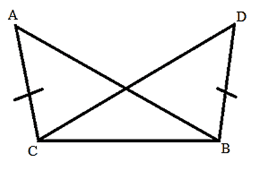### Course Features

• 728 Video Lectures
• Revision Notes
• Previous Year Papers
• Mind Map
• Study Planner
• NCERT Solutions
• Discussion Forum
• Test paper with Video Solution